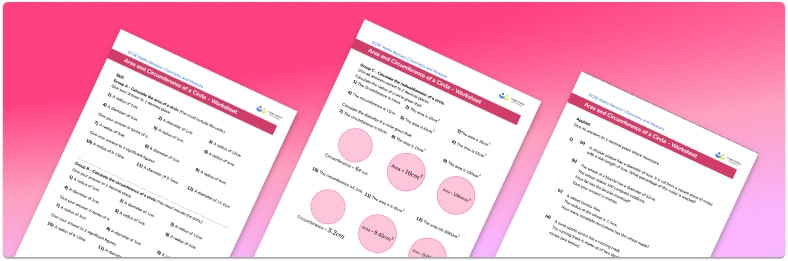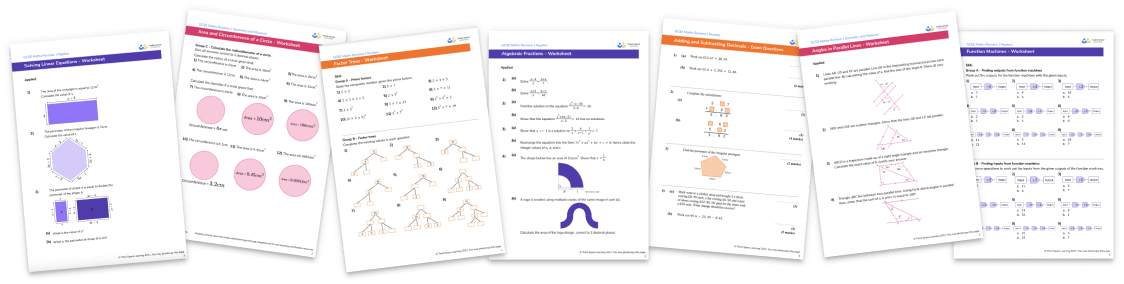# Area And Circumference Of A Circle WorksheetHelp your students prepare for their Maths GCSE with this free area and circumference of a circle worksheet of 45 questions and answers

• Section 1 of the printable area and circumference of a circle worksheet contains 36 skills-based questions, in 3 groups to support differentiation
• Section 2 contains 5 applied area and circumference of a circle questions with a mix of worded problems and deeper problem solving questions
• Section 3 contains 6 foundation and higher level GCSE exam style questions
• Answers and a mark scheme for all questions are provided
• Questions follow variation theory with plenty of opportunities for students to work independently at their own level
• All questions created by fully qualified expert secondary maths teachers
• Suitable for GCSE maths revision for AQA, OCR and Edexcel exam boards
• Other worksheets to accompany the circumference and area of circle worksheet, including area of compound shapes and graphing circles, are available

• This field is for validation purposes and should be left unchanged.

You can unsubscribe at any time (each email we send will contain an easy way to unsubscribe). To find out more about how we use your data, see our privacy policy.

### Area and circumference of a circle at a glance

The formula for the circumference of a circle is equal to pi times D, where D is the diameter of the circle. If the diameter is given we just multiply it by π to find the circumference of the circle. If the radius is given we need to multiply it by 2 to find the diameter of the circle, then proceed as above.

The formula for the area of a circle is  equal to πr², where r is the radius of the circle. If the radius is given, we need to square it and multiply it by π to find the area of the circle. If the diameter of a circle is given instead of its radius, we need to divide the diameter by 2 to find the radius, then proceed as above. If the area of a circle is given, students may be asked to work backwards to find the length of the radius. Here, we need to divide by and find the square root.

Students may sometimes be asked to leave their answer in terms of pi; if this is not the case they will need to round the decimal answer to a given number of decimal places or significant figures. Converting between metric units is also important.

This topic can be extended further to the area of a semicircle and the area of other fractions of a circle. We can also use the circumference formula to calculate an arc length. Word problems may also require students to apply knowledge of compound shapes.

Looking forward, students can then progress to additional circles, sectors and arcs worksheets and other geometry worksheets, for example an angles in polygons worksheet or area and circumference of a circle worksheet.For more teaching and learning support on Geometry our GCSE maths lessons provide step by step support for all GCSE maths concepts.

## Do you have KS4 students who need more focused attention to succeed at GCSE?There will be students in your class who require individual attention to help them succeed in their maths GCSEs. In a class of 30, it’s not always easy to provide.

Help your students feel confident with exam-style questions and the strategies they’ll need to answer them correctly with our dedicated GCSE maths revision programme.

Lessons are selected to provide support where each student needs it most, and specially-trained GCSE maths tutors adapt the pitch and pace of each lesson. This ensures a personalised revision programme that raises grades and boosts confidence.

Find out more